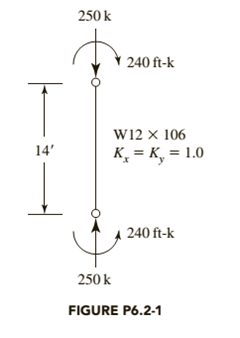Chapter 6, Problem 6.2.1P### Steel Design (Activate Learning wi...

6th Edition
Segui + 1 other
ISBN: 9781337094740

#### Solutions

Chapter
Section### Steel Design (Activate Learning wi...

6th Edition
Segui + 1 other
ISBN: 9781337094740
Textbook Problem

# Determine whether the given member satisfies the appropriate AISC interaction equation. Do not consider moment amplification. The loads are 50 % dead load and 50 % live load. Bending is about the x axis, and the steel is ASTM A992.a. Use LRFD.b. Use ASD.To determine

(a)

The satisfaction of AISC interaction equation using LRFD.

The member satisfies the AISC interaction equation.

Explanation

Given:

The load is 250kips.

The length of member is 14feet.

The value of kx and ky are 1.0 and 1.0.

The flexural load is 240ftkips.

Concept Used:

Write the LRFD interaction equation.

PuϕcPn+89(MuxϕbMnx+MuyϕbMny)1.0 ...... (I)

Here, the factored load is Pu, the axial compressive design strength is ϕcP, the flexural load about x and y-axis are Mux and Muy and the nominal flexural strength about x and y-axis are ϕbMnx and ϕbMny.

Calculation:

Calculate the factored load.

Pu=1.2(DL)+1.6(LL) ...... (II)

Here, the dead load is DL and the live load is LL.

Substitute 125kips for DL and 125kips for LL in Equation (II).

Pu=1.2(125kips)+1.6(125kips)=150kips+200kips=350kips

Calculate the effective length of the member.

Le=ky×L ...... (III)

Here, the unsupported length is L and the effective length factor is ky.

Substitute 14ft for L and 1.0 for ky in Equation (III).

Le=1.0×14ft=14ft

Calculate the axial compressive design strength.

From the manual table, the axial compressive design strength of a W12×106 with fy=50ksi and Le=14ft is 1130kips.

Calculate the nominal flexural strength about x-axis.

From the design table, calculate the nominal flexural strength about x-axis by using Cb=1.0 and Lb=14ft.

ϕbMnx=597ftkips

Mux=1.2(MD)+1.6(ML) ...... (IV)

Here, the flexural dead load is MD and the flexural live load is ML.

Substitute 120ftkips for MD and 120ftkips for ML in Equation (IV).

Mux=1.2(120ftkips)+1.6(120ftkips)=144ftkips+192ftkips=336ftkips

There is no bending about y-axis, therefore ϕbMny=0ftkips and Muy=0ftkips.

Write the equation to calculate the controlling interaction formula.

r=PuϕcPn ...... (V)

Substitute 44kips for Pu and 1130kips for ϕcPn in Equation (V).

r=350kips1130kips=0.3097

The value is greater than 0.2.

Calculate the LRFD interaction equation.

Substitute 350kips for Pu, 1130kips for ϕcPn, 336ftkips for Mux, 0ftkips for Muy, 597ftkips for ϕbMnx and 0ftkips for ϕbMny in Equation (I).

350kips1130kips+89(336ftkips597ftkips+0ftkips0ftkips)1.00.3097+89(0.5628)1.00.3097+0.50021.00.80991.0

The interaction equation is satisfied.

Conclusion:

Therefore, the interaction equation is satisfied with the AISD interaction equation.

To determine

(b)

The satisfaction of AISC interaction equation using ASD.

The member satisfies the AISC interaction equation.

Explanation

Concept Used:

Write the ASD interaction equation.

PuPnΩc+89(MuxMnxΩc+MuyMnyΩc)1.0 ...... (VI)

Here, the factored load is Pu, the allowed compressive strength is PnΩc, the flexural load about x and y-axis are Mux and Muy and the nominal flexural strength about x and y-axis are MnxΩc and MnyΩc.

Calculation:

Calculate the factored load.

Pu=DL+LL ...... (VII)

Here, the dead load is DL and the live load is LL.

Substitute 125kips for DL and 125kips for LL in Equation (VII).

Pu=125kips+125kips=250kips

Calculate the allowed compressive strength.

From the manual table, the allowed compressive strength of a W12×106 with fy=50ksi and Le=14f is 755kips.

Calculate the nominal flexural strength about x-axis.

From the design table, calculate the nominal flexural strength about x-axis by using Cb=1.0 and Lb=14f.

MnxΩc=398ftkips

Mux=MD+ML ...... (VIII)

Here, the flexural dead load is MD and the flexural live load is ML.

Substitute 120ftkips for MD and 120ftkips for ML in Equation (VIII).

Mux=120ftkips+120ftkips=240ftkips

There is no bending about y-axis, therefore MnyΩc=0ftkips and Muy=0ftkips.

Write the equation to calculate the controlling interaction formula.

r=PuPnΩc ...... (IX)

Substitute 250kips for Pu and 755kips for PnΩc in Equation (IX).

r=250kips755kips=0.331

The value is greater than 0.2.

Calculate the ASD interaction equation.

Substitute 250kips for Pu, 755kips for PnΩc, 240ftkips for Mux, 0ftkips for Muy, 398ftkips for MnxΩc and 0ftkips for MnyΩc in Equation (VI).

250kips755kips+89(240ftkips398ftkips+0ftkips0ftkips)1.00.3311+89(0.6030)1.00.3311+0.5361.00.86711.0

The interaction equation is satisfied.

Conclusion:

Therefore, the interaction equation is satisfied with the ASD interaction equation.

#### The Solution to Your Study Problems

Bartleby provides explanations to thousands of textbook problems written by our experts, many with advanced degrees!

Get Started

## Additional Engineering Solutions

#### Convert P = 5.00 atm into Pa, bar, and psia.

Fundamentals of Chemical Engineering Thermodynamics (MindTap Course List)

#### What strategies e Wal-Mart and Loweâ€™s using to gain more online customers?

Systems Analysis and Design (Shelly Cashman Series) (MindTap Course List)

Find all the answers to your study problems with bartleby.
Textbook solutions plus Q&A. Get As ASAP arrow_forward